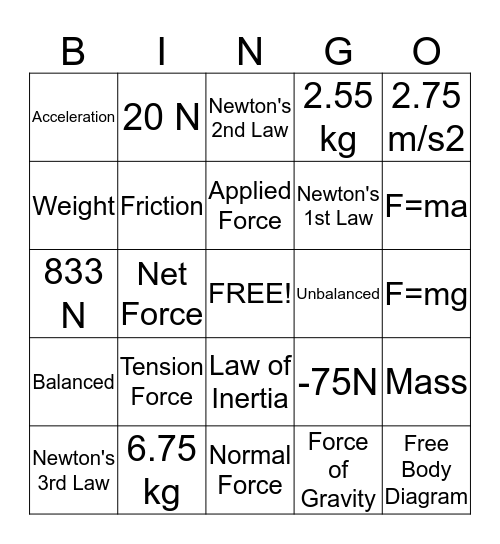# Forces BingoThis bingo card has a free space and 24 words: Tension Force, 6.75 kg, Applied Force, Newton's 3rd Law, 2.75 m/s2, Acceleration, Weight, 20 N, Unbalanced, Law of Inertia, Newton's 1st Law, 833 N, -75N, Mass, Friction, Force of Gravity, F=mg, Newton's 2nd Law, F=ma, Net Force, Free Body Diagram, Balanced, 2.55 kg and Normal Force.

## Play Online